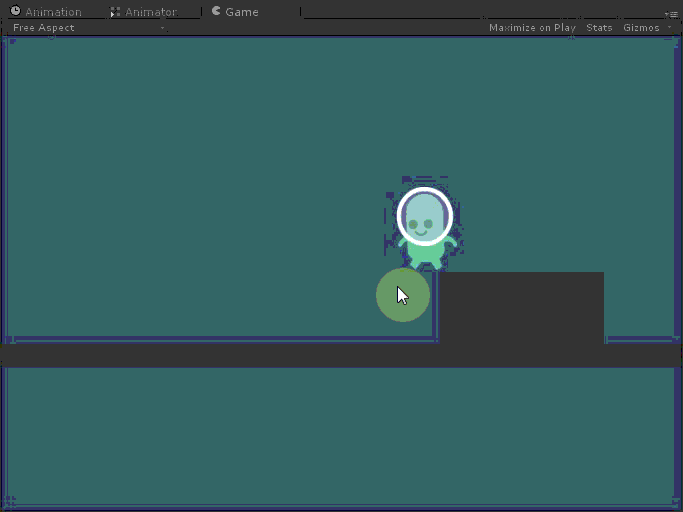# jump and check ground problem!

How do I solve this problem?When a player is placed in a corner , player can’t jump!!!

my script is:

``````using UnityEngine;
using System.Collections;

public class asli : MonoBehaviour {
public Animator anim;
public bool jump = false;				// Condition for whether the player should jump.
public float jumpForce = 1000f;			// Amount of force added when the player jumps.
private Transform groundCheck;			// A position marking where to check if the player is grounded.
private bool grounded = false;			// Whether or not the player is grounded.
public float speed=2;

void Start () {
anim=gameObject.GetComponent<Animator>();
groundCheck = transform.Find("groundCheck");
}

// Update is called once per frame
void Update () {

grounded = Physics2D.Linecast(transform.position, groundCheck.position, 1 << LayerMask.NameToLayer("Ground"));
if(grounded==true){	anim.SetBool("paresh",false);}else{anim.SetBool("paresh",true);}
if(Input.GetButtonDown("Jump") && grounded)
jump = true;
}
void FixedUpdate ()
{

anim.SetFloat("M",Mathf.Abs(Input.GetAxisRaw("Horizontal")));
if(Input.GetAxisRaw("Horizontal")>0)
{

transform.Translate(Vector3.right*speed*Time.deltaTime);
transform.eulerAngles=new Vector2(0,0);
}
if(Input.GetAxisRaw("Horizontal")<0)
{

transform.Translate(Vector3.right*speed*Time.deltaTime);
transform.eulerAngles=new Vector2(0,180);
}

// If the player should jump...
if(jump)
{

// Add a vertical force to the player.

// Make sure the player can't jump again until the jump conditions from Update are satisfied.
jump = false;
}
}
void OnCollisionExit2D(Collision2D coll) {
if (coll.gameObject.tag == "ground")
grounded=true;

}
}
``````

My guess is your linecast is failing when you hang over the edge. You can probably figure it out by debugging the transform.position value and groundCheck.position value when you hang over the edge.

Use a sphereCast instead of linecast, and problem solved, make sure the radius of the sphere match with the width of your character